# Control Statement Programs in C++

## Control Statement Programs in C++:

In this article, I am going to discuss Control Statement Programs in C++ with Examples. Please read our previous article where we discussed Switch Case Statements in C++ with Examples.

##### Bill Amount Program:
```#include <iostream>
using namespace std;
int main()
{
float billAmount;
float discount = 0.0;

cout << "Enter Bill Amount:";
cin >> billAmount;

if (billAmount >= 500)
discount = billAmount * 20 / 100;
else if (billAmount >= 100 && billAmount < 500)
discount = billAmount * 10 / 100;

cout << "Bill Amount is:" << billAmount << endl;
cout << "Discount is :" << discount << endl;
cout << "Discounted Amount is:" << billAmount - discount << endl;

return 0;
}
```
###### Output: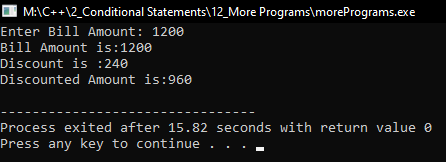##### Leap Year Program:
```#include <iostream>
using namespace std;

int main()
{
int year;

cout << "Enter a year: ";
cin >> year;

if (year % 4 == 0)
{
if (year % 100 == 0)
{
if (year % 400 == 0)
cout << year << " is a leap year.";
else
cout << year << " is not a leap year.";
}
else
cout << year << " is a leap year.";
}
else
cout << year << " is not a leap year.";
return 0;
}
```
###### Output: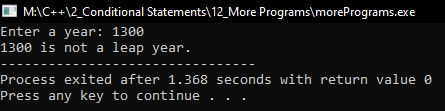##### Program to check given number is positive or negative:
```#include <iostream>
using namespace std;
int main()
{
float x;
cout << "Enter a Number: ";
cin >> x;

if (x > 0)
cout << "Number is Positive" << endl;
else
cout << "Number is negative" << endl;

return 0;
}
```
###### Output: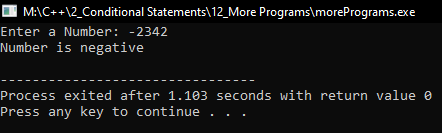##### Program to check given number is odd or even:
```#include <iostream>
using namespace std;
int main()
{
int x;
cout << "Enter a Number: ";
cin >> x;

if (x % 2 == 0)
cout << "Number is Even" << endl;
else
cout << "Number is Odd" << endl;

return 0;
}
```
###### Output: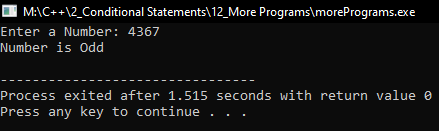##### Program to print Month’s Name:
```#include<iostream>
using namespace std;
int main()
{
int month;
cout << "Enter the Month's number :";
cin >> month;

switch (month)
{
case 1:
cout << "January";
break;
case 2:
cout << "Febrauary";
break;
case 3:
cout << "March";
break;
case 4:
cout << "April";
break;
case 5:
cout << "May";
break;
case 6:
cout << "June";
break;
case 7:
cout << "July";
break;
case 8:
cout << "August";
break;
case 9:
cout << "September";
break;
case 10:
cout << "October";
break;
case 11:
cout << "November";
break;
case 12:
cout << "December";
break;
}
return 0;
}
```
###### Output: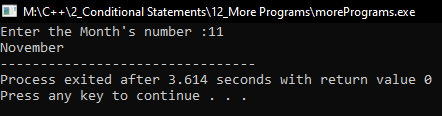##### Program to print Digits in words:
```#include<iostream>
using namespace std;
int main ()
{
char digit;
cout << "Enter a digit: ";
cin >> digit;

switch (digit)
{
case '0':
cout << "Zero";
break;
case '1':
cout << "One";
break;
case '2':
cout << "Two";
break;
case '3':
cout << "Three";
break;
case '4':
cout << "Four";
break;
case '5':
cout << "Five";
break;
case '6':
cout << "Six";
break;
case '7':
cout << "Seven";
break;
case '8':
cout << "Eight";
break;
case '9':
cout << "Nine";
break;
}
return 0;
}
```
###### Output: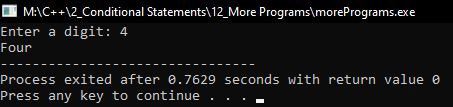Here, in this article, I try to explain Control Statement Programs in C++ with Examples and I hope you enjoy this Control Statement Programs in C++ with Examples article.